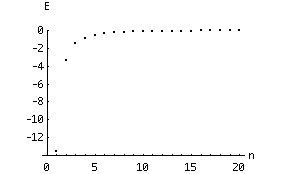# Bohr Model of the Atom

For the career physicist, study of the atom takes many years and still leads to new and interesting discoveries. For while we have an excellent set of models, which are capable of amazingly accurate predictions, we still cannot say that we KNOW what atoms are all about. We will barely scratch the surface of atomic physics, and we will see more of physics' mysteries.

While the Bohr Model of the atom is primarily of historical interest to the working physicist, it displays the essential qualities of the atom as intuitively as possible.

We start with a model of the atom which looks like a solar system: in place of the sun we have the positively charged nucleus, and instead of planets we have negatively charged electrons (see the next chapter). For the moment, we can imagine the electrons moving in circular (or elliptical) paths around the nucleus, but not constrained to lie in a plane, as most planets do. The centripetal acceleration is provided by the Coulomb attraction of the nucleus for the electrons. By equating the magnitude of the Coulomb force to the centripetal force, we can relate the radius of the orbit to the electron velocity:

m v2 / r = Z e2 / 4 p e0 r2,

or

r = Z e2 / 4 p e0 m v2,

where Z is the " atomic number" (the number of protons in the nucleus) and m is the mass of an electron.

There is only one problem with this model. The electrons are accelerating (due to their circular orbits), and accelerating charges radiate energy (the reasons for this are well beyond the scope of this text; trust us, it happens). Therefore a charged particle should lose energy and quickly spiral in to the nucleus in just a few orbits. To "solve" this problem, Bohr imposed a "quantization" condition: constrain the angular momentum to be an integer times Planck's constant over 2p. This prevents the electron from losing energy continuously; instead it must lose (or gain) energy in jumps (quanta). By imposing this condition on the electron, Bohr has not solved the problem, for we now have an inconsistent model: an accelerating charged particle which cannot lose angular momentum (or energy) continuously. The solution is actually to abandon the "classical" concept of a point particle with a well-defined orbit (see the next section). But Bohr's model leads to a decent agreement with experimental data (see Section C) and, as we shall see at the end of Chapter 9, it provides us with some clues to the nature of the electron itself.

Bohr's condition, then, gives us a second relationship between r and v:

m v r = n h / 2p.

We can solve this system to obtain the orbital radius and velocity for the nth orbit:

rn = n2 a0 / Z

and

vn = Z e2 / 2e0 n h,

where

a0 = e0 h2 / p m e2

and n is an integer (ranging from 1 to infinity). By equating the energy of an electron to the sum of its kinetic and potential energies:

E = 1/2 m v2 - Z e2 / 4 p e0 r ,

we obtain

En = - E0 Z2 / n2,

where E0 (= m e4 / 8 e02 h2) has a value of 2.18 x 10 - 18 J. So, as promised in the last chapter, the energy of an electron "bound" in an atom is quantized. Due to the orders of magnitude involved, it is more convenient to express energies of electrons in "electron Volts": 1 eV is 1.6 x 10 - 19 J, and E0 is then 13.6 eV.

Note that the energies of the bound electrons are negative. This reflects our choice of zero potential energy as corresponding to a free electron with zero velocity. As n becomes very large, the difference between two succeeding energy "levels" becomes vanishingly small:In the limit of n approaching infinity, the energy "spectrum" (set of levels) becomes continuous. This is called the "classical limit" , since classical energies are not quantized but can be any real number. A basic tenet of the "quantum theory" is that as n approaches infinity, the equations (ie., for energy) should become equivalent to their classical (macroscopic) counterparts. This idea is called the "correspondence principle" .

The next section is about quantum numbers and the periodic table.

If you have stumbled on this page, and the equations look funny (or you just want to know where you are!), see the College Physics for Students of Biology and Chemistry home page.